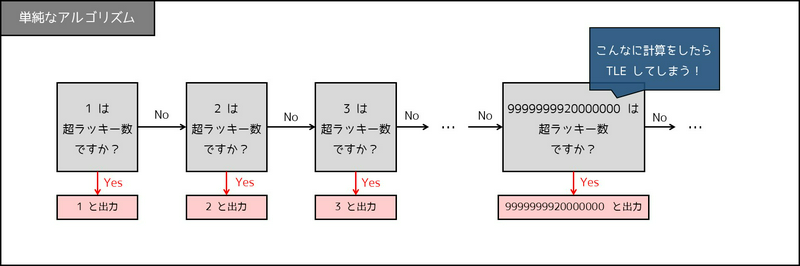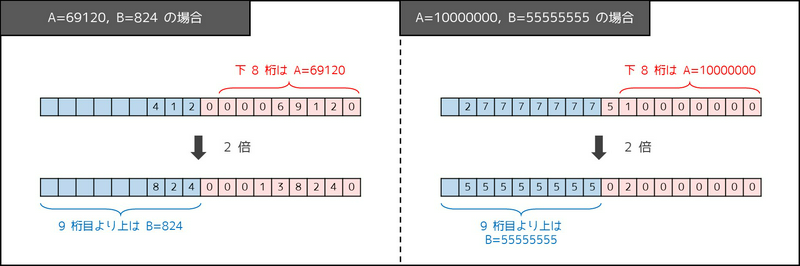Contest Duration: - (local time) (120 minutes) Back to Home

## A - Two Lucky Numbers Editorial by evima

If you are new to programming and at a loss, go to practice contest and try Problem A, with sample solutions in each language.

• 1. Naive algorithm
• 2. Intended solution
• 3. Proof
• 4. Hints for coming up with the solution
• 5. Source codes

To see just the solution, go to 2. Intended solution.

### 1. Naive algorithm

Here is a simple algorithm to solve this problem:

• Determine if $$x=1$$ is super-lucky. (If yes, print 1 and terminate.)
• Determine if $$x=2$$ is super-lucky. (If yes, print 2 and terminate.)
• Determine if $$x=3$$ is super-lucky. (If yes, print 3 and terminate.)
• And so on, until encountering a super-lucky number for the first time.

However, this can take long. For example, if $$A=99999999, B=40000000$$, the smallest super-lucky number is 9999999920000000, with 16 digits. Computers are not infinitely fast, and a solution that performs more than $$10^8 \sim 10^9$$ calculations (the number depends on programming language) will get a Time Limit Exceeded verdict. (Source Code in C++)

Let us try a different approach.### 2. Intended solution

It turns out that, under the Constraints, $$500,000,000 \times B + A$$ is always super-lucky.

Thus, the following program (C++) that prints this value will get accepted.

See 5. Source codes for codes in Python, Java, C.

#include <iostream>
#include <string>
using namespace std;

int main() {
long long A, B; // declare variables A, B of type long long
cin >> A >> B; // receive integers A, B
cout << 500000000LL * B + A << endl; // LL stands for long long
return 0;
}


### 3. Proof

Here is the proof of the solution.

Let $$X=500,000,000 \times B+A$$. Then, we have $$2X = 10^9 \times B + 2A$$, and the following holds.

• The lowest $$8$$ digits of $$X$$ form $$A$$, satisfying the first condition ($$X$$ contains $$A$$).
• Since $$A \leq 99999999$$, the lowest $$9$$ digits of $$2X$$ form $$2A$$, and the digits above them form $$B$$, satisfying the second condition ($$2X$$ contains $$B$$).

For reference, here are the values of $$X$$ and $$2X$$ in some cases.### 4. Hints for coming up with the solution

In a problem that asks you to “construct” one solution, it is typically helpful to consider just part of the given conditions and then modify the provisional solution a bit. In this problem, it allows us to reach the intended solution as follows.

Step 1: Consider just the first condition

What integer $$x$$ satisfies the first condition ($$x$$ contains $$A$$)? Obviously, $$x=A$$ does.

Step 2: Possible modification

Now, consider modifying $$x$$ a bit so that it still satisfies the condition. We can see that modifying the part above the lowest $$9$$ digits does not break it. (For example, when $$A=1234567$$, changing it to $$x=1001234567$$ or $$x=869001234567$$ is fine.)

Step 3. Add the second condition

Now, consider making the upper digits of $$2x$$ contain $$B$$. It should be possible to derive $$x=500,000,000 \times B+A$$.

This pattern of thought can also be used in other problems such as ARC 117 A - God Sequence.

posted:
last update: